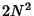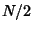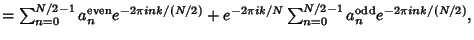## Fast Fourier Transform

The fast Fourier transform (FFT) is a Discrete Fourier Transform Algorithm which reduces the number of computations needed forpoints fromto, where Lg is the base-2 Logarithm. If the function to be transformed is not harmonically related to the sampling frequency, the response of an FFT looks like a Sinc Function (although the integrated Power is still correct). Aliasing (Leakage) can be reduced by Apodization using a Tapering Function. However, Aliasing reduction is at the expense of broadening the spectral response.

FFTs were first discussed by Cooley and Tukey (1965), although Gaußhad actually described the critical factorization step as early as 1805 (Gergkand 1969, Strang 1993). A Discrete Fourier Transform can be computed using an FFT by means of the Danielson-Lanczos Lemma if the number of pointsis a Power of two. If the number of pointsis not a Power of two, a transform can be performed on sets of points corresponding to the prime factors ofwhich is slightly degraded in speed. An efficient real Fourier transform algorithm or a fast Hartley Transform (Bracewell 1965) gives a further increase in speed by approximately a factor of two. Base-4 and base-8 fast Fourier transforms use optimized code, and can be 20-30% faster than base-2 fast Fourier transforms. Prime factorization is slow when the factors are large, but discrete Fourier transforms can be made fast for, 3, 4, 5, 7, 8, 11, 13, and 16 using the Winograd Transform Algorithm (Press et al. 1992, pp. 412-413, Arndt).

Fast Fourier transform algorithms generally fall into two classes: decimation in time, and decimation in frequency. The Cooley-Tukey FFT Algorithm first rearranges the input elements in bit-reversed order, then builds the output transform (decimation in time). The basic idea is to break up a transform of lengthinto two transforms of lengthusing the identitysometimes called the Danielson-Lanczos Lemma. The easiest way to visualize this procedure is perhaps via the Fourier Matrix.

The Sande-Tukey Algorithm (Stoer and Bulirsch 1980) first transforms, then rearranges the output values (decimation in frequency).

See also Danielson-Lanczos Lemma, Discrete Fourier Transform, Fourier Matrix, Fourier Transform, Hartley Transform, Number Theoretic Transform, Winograd Transform

References

Arndt, J. FFT Code and Related Stuff.'' http://www.jjj.de//fxt/.

Bell Laboratories. Netlib FFTPack.'' http://netlib.bell-labs.com/netlib/fftpack/.

Blahut, R. E. Fast Algorithms for Digital Signal Processing. New York: Addison-Wesley, 1984.

Bracewell, R. The Fourier Transform and Its Applications. New York: McGraw-Hill, 1965.

Brigham, E. O. The Fast Fourier Transform and Applications. Englewood Cliffs, NJ: Prentice Hall, 1988.

Cooley, J. W. and Tukey, O. W. An Algorithm for the Machine Calculation of Complex Fourier Series.'' Math. Comput. 19, 297-301, 1965.

Duhamel, P. and Vetterli, M. Fast Fourier Transforms: A Tutorial Review.'' Signal Processing 19, 259-299, 1990.

Gergkand, G. D. A Guided Tour of the Fast Fourier Transform.'' IEEE Spectrum, pp. 41-52, July 1969.

Lipson, J. D. Elements of Algebra and Algebraic Computing. Reading, MA: Addison-Wesley, 1981.

Nussbaumer, H. J. Fast Fourier Transform and Convolution Algorithms, 2nd ed. New York: Springer-Verlag, 1982.

Papoulis, A. The Fourier Integral and its Applications. New York: McGraw-Hill, 1962.

Press, W. H.; Flannery, B. P.; Teukolsky, S. A.; and Vetterling, W. T. Fast Fourier Transform.'' Ch. 12 in Numerical Recipes in FORTRAN: The Art of Scientific Computing, 2nd ed. Cambridge, England: Cambridge University Press, pp. 490-529, 1992.

Stoer, J. and Bulirsch, R. Introduction to Numerical Analysis. New York: Springer-Verlag, 1980.

Strang, G. Wavelet Transforms Versus Fourier Transforms.'' Bull. Amer. Math. Soc. 28, 288-305, 1993.

Van Loan, C. Computational Frameworks for the Fast Fourier Transform. Philadelphia, PA: SIAM, 1992.

Walker, J. S. Fast Fourier Transform, 2nd ed. Boca Raton, FL: CRC Press, 1996.